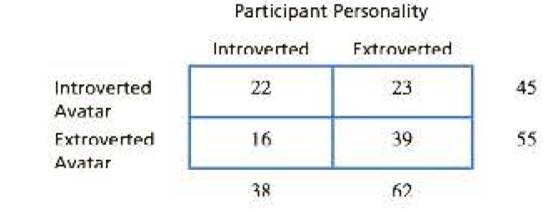Chapter 15, Problem 20PEssentials of Statistics for the B...

8th Edition
Frederick J Gravetter + 1 other
ISBN: 9781133956570

Solutions

Chapter
SectionEssentials of Statistics for the B...

8th Edition
Frederick J Gravetter + 1 other
ISBN: 9781133956570
Textbook Problem

A recent study indicates that people tend to select video game avatars with characteristics similar to those of their creators (Bélisle & Onur, 2010). Participants who had created avatars for a virtual community game completed a questionnaire about their personalities. An independent group of viewers examined the avatars and recorded their impressions of the avatars. One personality characteristic considered was introverted/ extroverted. The following table shows frequency distribution of personalities for participants and the avatars they created.a. Is there a significant relationship between the personalities of the participants and the personalities of their avatars? Test with α = 05. b. Compute the phi-coefficient to measure the size of the effect

a.

To determine

To check: Whether there is significant relationship between the personalities of the participants and the personalities of their avatars for the given question.

Explanation

Given info:

A sample of 100 students was involved in a study based on “the personalities for participants and their avatars”. Use α=0.05 to test the claim.

Calculations:

Step 1: Null Hypothesis and Alternate Hypothesis are:

H0: No significant relationship between the personalities of the participants and the personalities of their avatars.

H1: Significant relationship between the personalities of the participants and the personalities of their avatars

Step 2: For the given sample, degrees of freedom equals:

df=(R1)(C1)  where R equals number of rows and C equals columns=(21)(21)=1

With α=0.05 and df=1 , the critical value (CV)  is obtained from the χ2table as

χ2=3.841

Step 3: χ2statistics is calculated as:

χ2=(fofe)2fe

The formula to calculate expected frequency is:

fe=fcfrnwhere fr is row frequency and fc is column frequency

Substitute n=200 in the above formula and compute respective values of expected frequencies:

For the category “introverted avatar”, the expected frequencies are:

fe,introvert=38×45

b.

To determine

To Find: The value of phi-coefficient for the given question.

Still sussing out bartleby?

Check out a sample textbook solution.

See a sample solution

The Solution to Your Study Problems

Bartleby provides explanations to thousands of textbook problems written by our experts, many with advanced degrees!

Get Started

Given: AEBDEC AEDE Prove: AEBDEC

Elementary Geometry for College Students

In Exercises 110, find the graphical solution of each inequality. 4x3y24

Finite Mathematics for the Managerial, Life, and Social Sciences

True or False: The vector function is continuous at t = 3.

Study Guide for Stewart's Multivariable Calculus, 8th

True or False: If f(x) = g(x) for all x then f(x) = g(x).

Study Guide for Stewart's Single Variable Calculus: Early Transcendentals, 8th